Latest Banking jobs   »   Quantitative Aptitude Quiz For SBI Clerk...

# Quantitative Aptitude Quiz For SBI Clerk Mains 2022- 18th December

Directions (1-5): Find the wrong number in the following number series.

Q1. 810, 820, 832, 868, 1012, 1732, 6052
(a) 6052
(b) 810
(c) 868
(d) 832
(e) 1732

Q2. 1024, 350, 832, 508, 704, 604, 640
(a) 1024
(b) 640
(c) 704
(d) 350
(e) 508

Q3. 190, 210, 266, 358, 486, 646, 850
(a) 646
(b) 850
(c) 486
(d) 190
(e) 210

Q4. 15, 50, 160, 370, 709, 1208, 1904
(a) 15
(b) 50
(c) 370
(d) 1208
(e) 15

Q5. 120, 170, 251, 367, 522, 720, 990
(a) 120
(b) 990
(c) 522
(d) 367
(e) 251

Directions (6-10): In the following questions, two equations (I) and (II) are given. You have to solve both the equations and answer the following questions.

Q6. I. (x – 6) ² = x
II. (y – 5)² = 3y – 17
(a) x < y
(b) x > y
(c) x ≤ y
(d) x ≥ y
(e) x=y or no relation.

Q7. I. 24x² – 62x + 40 = 0
II. 15y²-59y + 56 = 0
(a) x < y
(b) x > y
(c) x ≤ y
(d) x ≥ y
(e) x=y or no relation.

Q8. I. 45x² + 116x + 63 = 0
II. 48y² + 110y + 63 = 0
(a) x < y
(b) x > y
(c) x ≤ y
(d) x ≥ y
(e) x=y or no relation.

Q9. I. x² – 16x + 63 = 0
II. y² – 11y + 28 = 0
(a) x < y
(b) x > y
(c) x ≤ y
(d) x ≥ y
(e) x=y or no relation.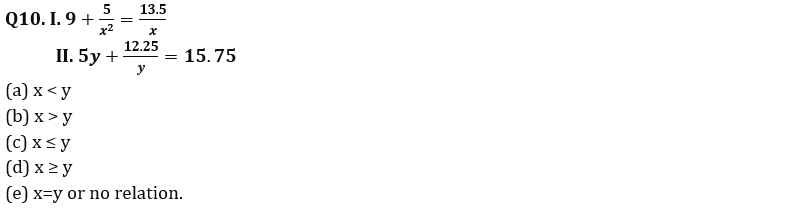Directions (11-15): In the following questions, two quantities (I) and (II) are given. You have to solve both the quantities and mark the appropriate answer.

Q11. Quantity I: Anurag invested Rs.3000 more than that of Veer, while ratio of investment period for Anurag and Veer is 5 : 6 respectively. If out of total profit of Rs.27360, Veer got Rs.12960, then find total investment done by Anurag.
Quantity II: Shivam and Deepak started a business by investing Rs.25000 and Rs.10000 respectively. At the end of the year, they decided to divide 60% of the total profit equally and rest in the investment ratio. If they had divided total profit in investment ratio, then Shivam will receive Rs.9000 more profit than that of he received actually, then find the difference between actual profit share of Shivam and that of Deepak?
(a) Quantity I < Quantity II
(b) Quantity I ≤ Quantity II
(c) Quantity I > Quantity II
(d) Quantity I ≥ Quantity II
(e) Quantity I = Quantity II or no relation.

Q12. Quantity I: Aman invested Rs.100000 at 16% p.a. The interest was compounded half yearly in the first year and in the second & third year it was compounded yearly. What will be the total interest received by man at the end of 3 years?
Quantity II: The simple interest received on a sum of Rs.2,30,000 at the rate of 23% p.a. in N years is Rs.4,23,200. Find the CI received on the same sum in (N/4) years at the rate of 11% p.a. compounded annually.
(a) Quantity I < Quantity II
(b) Quantity I ≤ Quantity II
(c) Quantity I > Quantity II
(d) Quantity I ≥ Quantity II
(e) Quantity I = Quantity II or no relation.

Q13. Quantity I: The ratio of speed of boat in still water to the speed of stream is 9 : 5. A boat takes total 7⅞ hours to travel 98 km each in upstream and in downstream. Find the speed of boat is still water.
Quantity II: Ratio of length of train – A to that of train – B is 3 : 4. Train – A crosses train – B in 48 seconds while running in same direction and train – B crosses a pole in 16 seconds. If train – A crosses a 520m long platform in 24 seconds, then find difference in speed of train – A & B. (in km/hr.)
(a) Quantity I < Quantity II
(b) Quantity I ≤ Quantity II
(c) Quantity I > Quantity II
(d) Quantity I ≥ Quantity II
(e) Quantity I = Quantity II or no relation.

Q14. Quantity I: Ratio of cost price of two articles – A & B is 5 : 6 respectively and shopkeeper marked article – A & B 40% and 25% above cost price respectively. If shopkeeper allows discount of 25% on A and 10% on B, then he get a total profit of Rs.300. Find the total cost price of article A & B.
Quantity II: Selling price of an article sold by Amit and Arun is same. Amit sold article at 20% profit and Arun sold at 25% loss. Amit calculated profit percent at cost price whereas Arun calculated loss percent at selling price. If total loss of Amit and Arun together is Rs.200. Find total cost price of Amit’s article and Arun’s article ?
(a) Quantity I < Quantity II
(b) Quantity I ≤ Quantity II
(c) Quantity I > Quantity II
(d) Quantity I ≥ Quantity II
(e) Quantity I = Quantity II or no relation.

Q15. Quantity I: x⁴ + y⁶ = 19721. If y = 5 and x & y are positive integers, then find x is what percent of y.
Quantity II: A is 20% less than B and B is 10% less than C. If ratio of A to D is 4 : 5, then find A & D together are what percent of C.
(a) Quantity I < Quantity II
(b) Quantity I ≤ Quantity II
(c) Quantity I > Quantity II
(d) Quantity I ≥ Quantity II
(e) Quantity I = Quantity II or no relation.

Solutions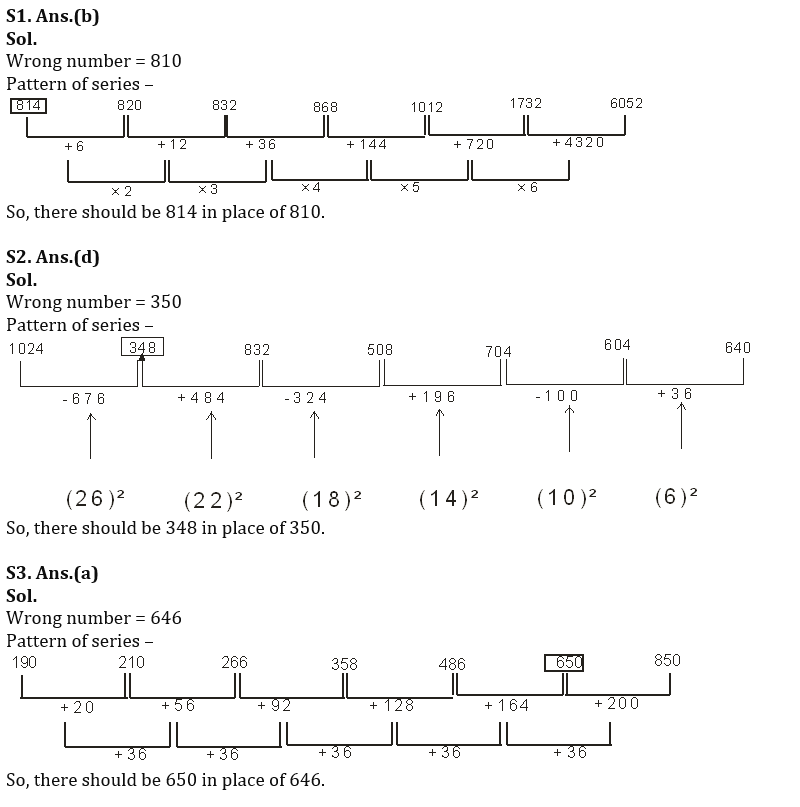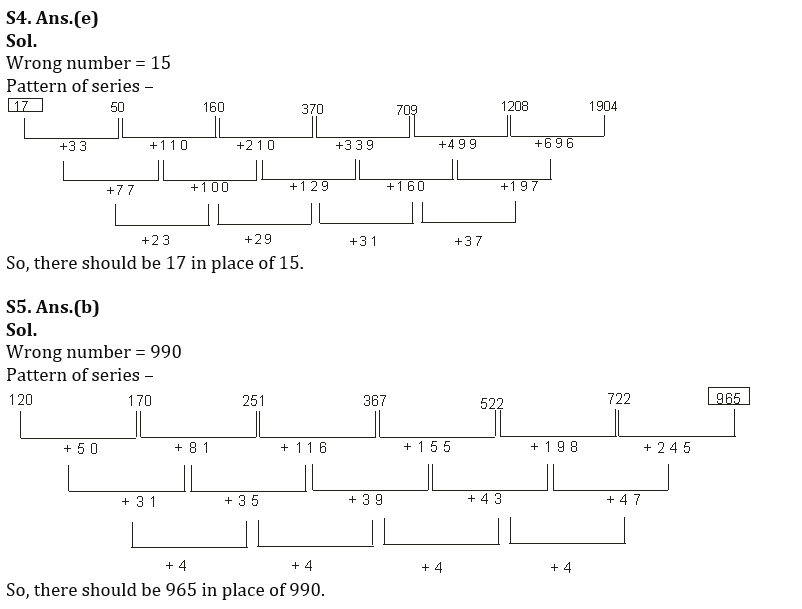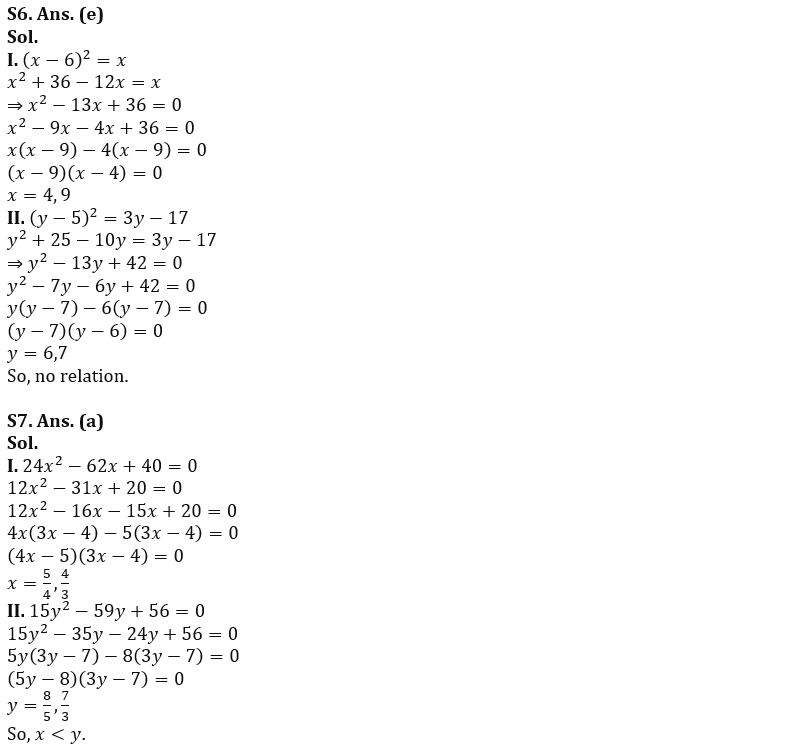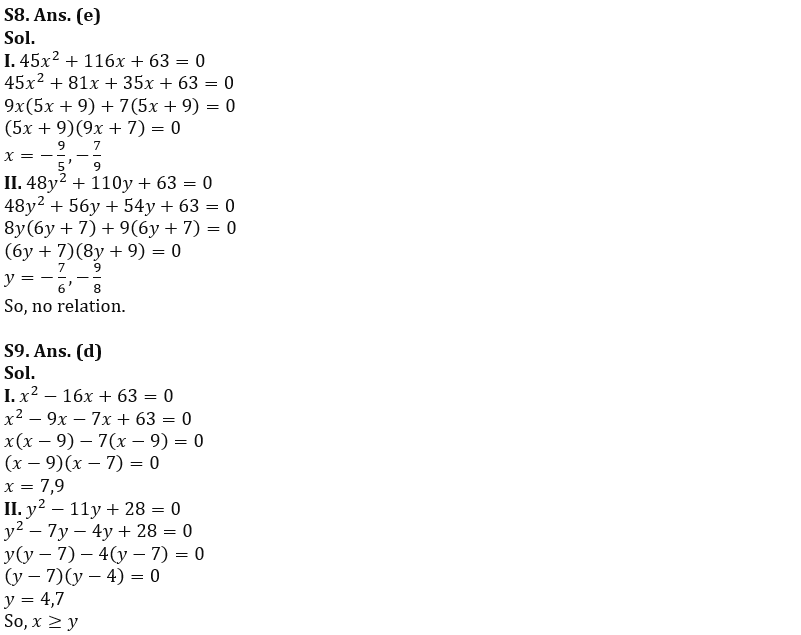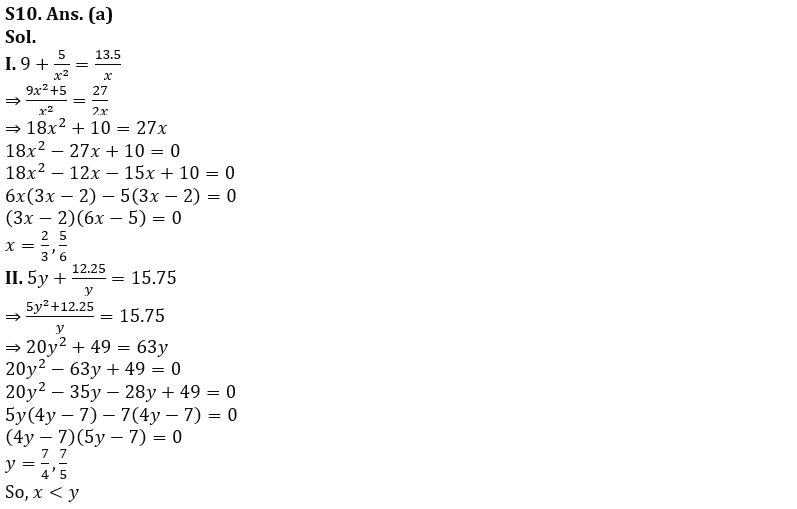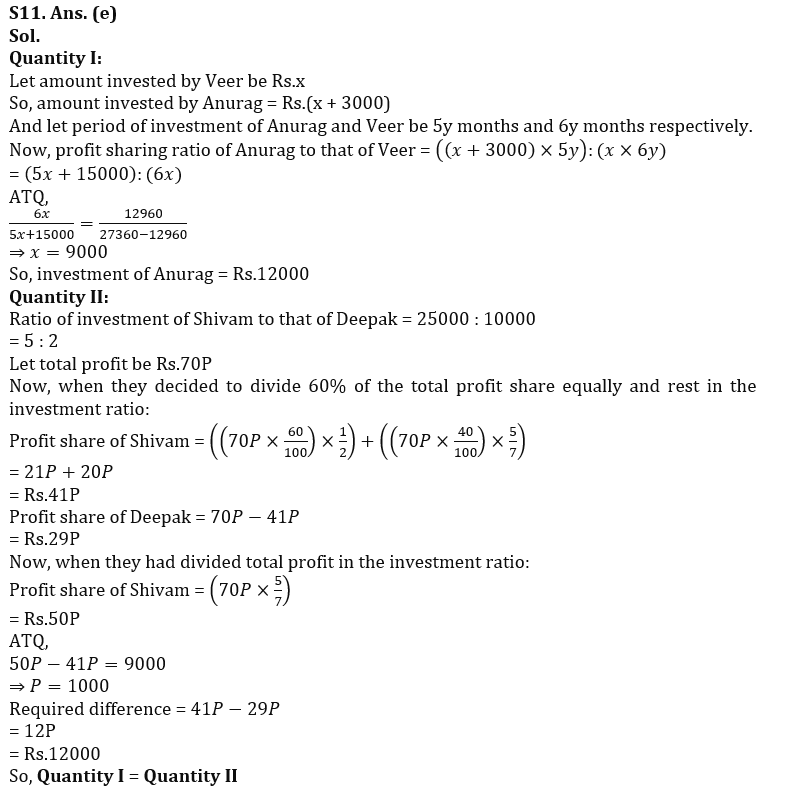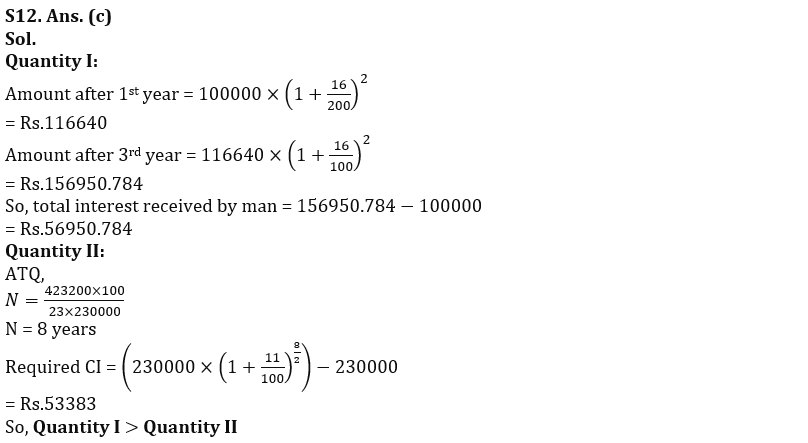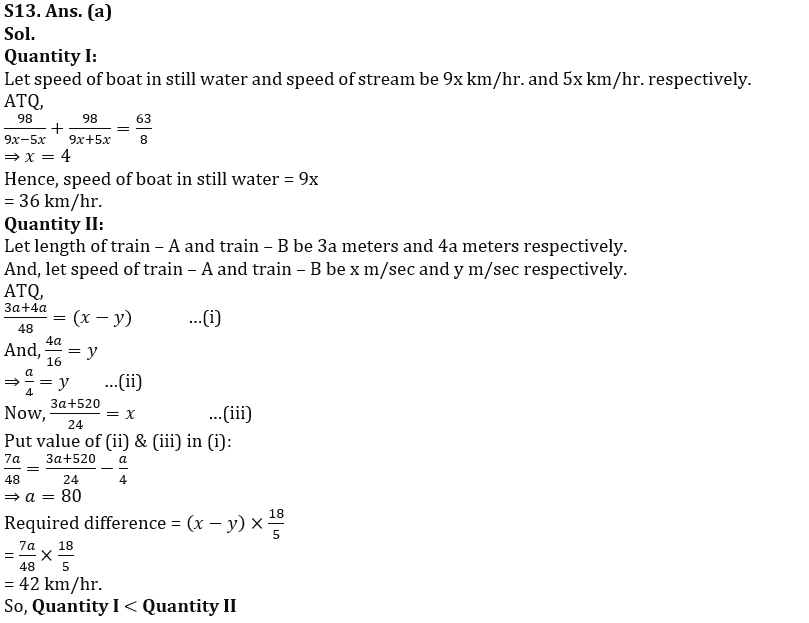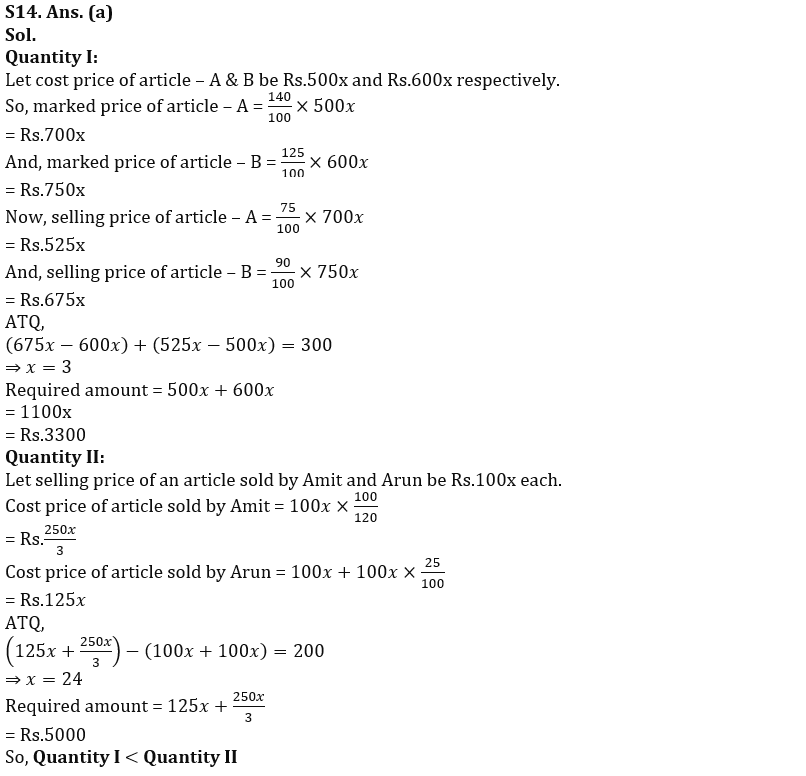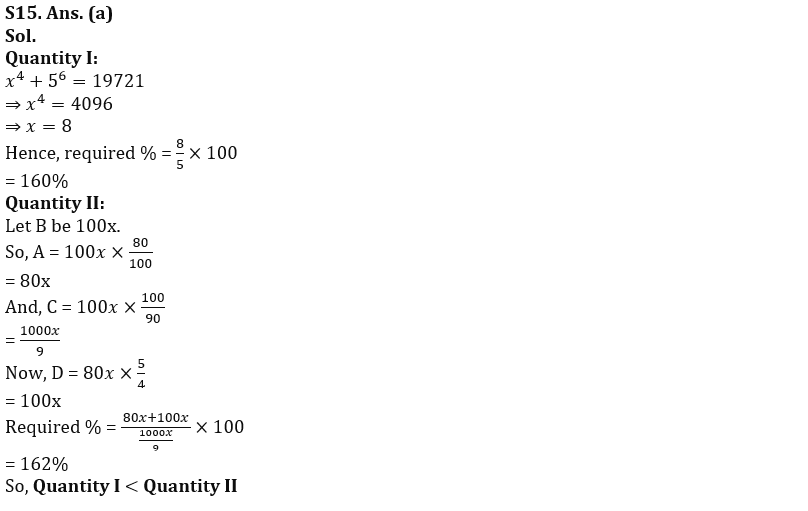#### Congratulations!Union Budget 2023-24: Free PDF